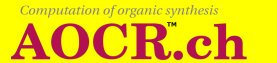# 2. The Computation Tool ( Web version )

The Computation Tool is a Java user friendly interface to the organic reaction computation program. A user must prepare with the Graphic Composer the input consisting of the formulas for the start and searched compounds and of the list of reaction step rules before beginning to compute with the tool.

### 2.1. Basic facts

The Computation Tool has two operating modes. These two modes can be selected from the MAIN list.

Both modes accept the same options.

In the start mode the tool begins computing with the formula saved with Save start under File in the Graphic Composer, applies the reaction step rules in the list built with Add rule to list under File and looks for the formula ofthe searched compounds given in the formula saved with Save searched under File.

The computation generates formulas from every formula in the generation following the given rules until a termination condition is fulfilled. A termination condition is for example given when the searched compounds are found in a generated formula. This is the case if they have a matching image in the generated formula.

For each reaction step rule in the list the program attempts to find the matching images in the formula of the generation where it is positioned at. Whenever it finds such an image it applies the specified bond or charge movements.

With the continue instruction the immediately previous generation is resumed at the previous position. The list of rules and the searched compounds are also taken from the previous execution. Hence a continue must follow an (error free) start or continue.

Figure 3.1Figure 3.1 above shows the generated sequence: The formulas n+m, n+m+1 are obtained from the formula n of generated compounds by the application of a rule at some position in formula n.